#Function Repository Resource:

# LinearFunctionQ

Determine whether an expression represents a linear function of a given set of variables

Contributed by: Paco Jain (Wolfram Research)
 ResourceFunction["LinearFunctionQ"][expr,var] returns True if expr is a linear function of the symbol var, and returns False otherwise. ResourceFunction["LinearFunctionQ"][expr,{var1,var2, …}] returns True if expr is a linear function of each of the symbols vari, considered together, and returns False otherwise.

## Details and Options

If expr is of the form Equal[lhs, rhs], ResourceFunction["LinearFunctionQ"][expr, ] is equivalent to ResourceFunction["LinearFunctionQ"][lhs - rhs, ]

## Examples

### Basic Examples

Test whether an expression represents a linear function of a given variable:

 In:=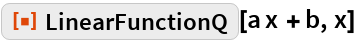Out=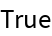In:=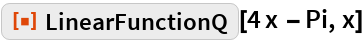Out=Test for linearity with respect to a list of variables:

 In:=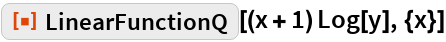Out=In:=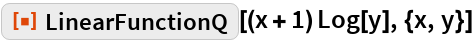Out=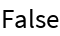In:=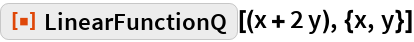Out=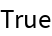### Scope

Variables listed in the second argument are considered together in the test for linearity:

 In:=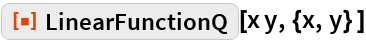Out=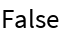### Possible Issues

Expressions that are constant with respect to a variable are not considered linear:

 In:=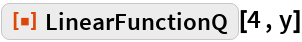Out=In:=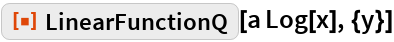Out=## Requirements

Wolfram Language 11.3 (March 2018) or above